Next: Experiments Up: Application to Bayesian Noisy Previous: Application to Bayesian Noisy

## Overview of the Bayesian ICA Algorithm

In , it is described how to use ensemble learning for the noisy ICA model. The posterior distribution is over all unknown parameters, including the mixing matrix A. In ensemble learning, a factorial approximationis fitted to the actual posterior distribution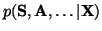by minimising the Kullback-Leibler information between them, i.e., the cost function which is minimized during learning is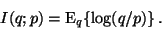The algorithm is computationally efficient when the approximation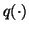of the posterior probability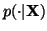is chosen to be factorial. This can be seen as an extension of the factorial EM-algorithm in , whereincluded only the posterior distribution of the sources. For further details, see for instance [6,8], where ensemble learning is applied to nonlinear ICA.

The ICA algorithm based on ensemble learning works in much the same way as EM-algorithm. First the distribution of the sources is computed by using the current estimate of the distribution of the other parameters. Then the distribution of the other parameters is computed using this distribution of the sources. The posterior distributions of the parameters are approximated by Gaussian distribution which means that for each element of the mixing matrix A, the posterior mean and variance is estimated. The modification will be applied to the posterior mean of the mixing matrix.

For each vector of the mixing matrix, the modified posterior mean will be the normalized difference between the posterior mean estimated from the original sources and the Gaussianized sources. The iteration is then repeated by estimating the posteriors of the sources again, using the new parameter distribution.

In practice, the algorithm is performed in deflatory manner, that is, the sources are extracted one by one. The mixtures are prewhitened and then the mixing matrix is estimated one column a at a time.

A heuristic stabilization is added to ensure convergence. This is achieved by updating the vector a to be a linear combination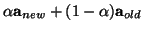. The coefficient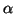is increased when consecutive corrections to a have a positive inner product which means that they do not change to opposite directions. Otherwise,is decreased.Next: Experiments Up: Application to Bayesian Noisy Previous: Application to Bayesian Noisy
Harri Lappalainen
2000-03-09